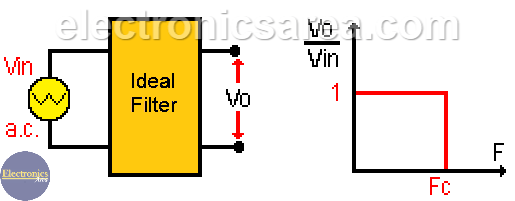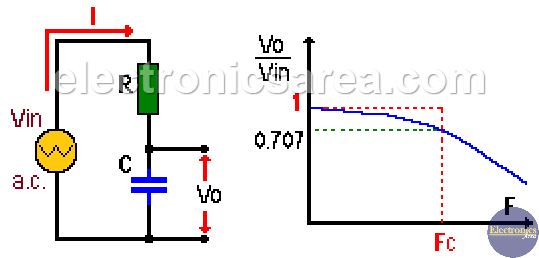# RC Low Pass Filter

An RC low pass filter is a circuit made by a resistor and a capacitor connected in series, as shown in the image below.

The RC low pass filter allows only the passage of frequencies below a particular frequency called cut-off frequency (Fc) and eliminates frequencies above this frequency. These RC low pass filters are not perfect so two analyzes are done.

An analysis with an ideal filter and another with a real filter. The unit of frequency is: Hertz or cycles per second.

## Ideal RC Low Pass FilterThe ideal RC low pass filter is a circuit made by a resistor and a capacitor in series, which allows the passage of frequencies below the cut-off frequency (Fc) and eliminates those that are above it. (see previous image).

## Real RC Low Pass FilterThe capacitive reactance (XC) changes with frequency. For high frequencies (above the cut-off frequency) the capacitive reactance XC is low, and the signals of these frequencies are attenuated.

In contrast, for low frequencies (below the cut-off frequency) the capacitive reactance is large, so that these frequencies are not affected or are affected very little by the filter.

Using Ohm’s law:

• Vin = I*Z = I*(R2 + XC2)1/2
• Vo = I*XC
• Vo = Vin/(1 + (2πf*RC)2)1/2

where:

• Z = Impedance
• π = Pi = 3.14159
• f = frequency in hertz
• R = resistance value
• C = capacitor value

The cut-off frequency is the frequency where the amplitude of the incoming signal falls to 70.7% (0.707) of its maximum value. This happens when XC = R. (capacitive reactance = resistance). If XC = R, the cut-off frequency is: Fc = 1/(2*π*R*C).

The frequency band below the cut-off frequency (Fc) is called the Pass Band, and the frequency band above Fc is called Stop Band.

## Phase angle of a RC low pass filter

The phase angle of a RC low pass filter as a function of frequency is obtained using the formula: ψ = tan-1 (R / XC).

Note: (  )1/2 = square root.

• 2
•
•
•
•
•
•
•
•
2
Shares
•
2
Shares
• 2
•
•
•
•
•
•
•